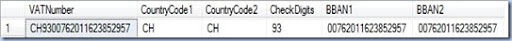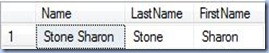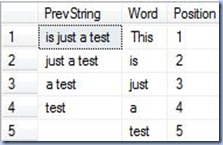## 24 February 2011

### SQL Reloaded: Pulling the Strings of SQL Server VI (Subparts of a String)

No matter how normalized a database is, there will always be the need to encode multiple semantic entities in a string, needing thus to extract them later. For example data like the Street Name and Number, or the Country Phone Prefix and Phone Number, the First and the Last Name, etc. Another frequent scenario is the misuse of a long-sized string data types to store multiple delimited attributes or simply a whole text like a Comment or Description. The easiest scenarios to deal with are the ones when you know the rules behind your encoding, preferably dealing with a fix length encoding or only a given delimiter. An example of fix length encoding is the IBAN Number, the country specific VAT Number or any other artificial constructed/standardized encoding. According to Wikipedia, IBAN (International Bank Account) Number consists of  ISO 3166-1 alpha-2 country code, followed by two check digits that are calculated using a mod-97 technique, and Basic Bank Account Number (BBAN) with up to thirty alphanumeric characters. Taking the IBAN example provided for Switzerland, it could be stored as “CH93 0076 2011 6238 5295 7” or “CH9300762011623852957”, in either case when the country is not provided explicitly it would be interesting to extract it from the IBAN together with the BBAN. How would we do that?

Many of the programming languages I worked with provide a function for extracting a substring from a string - Mid (VB/VBScript), Substring in C# Substr in Oracle, etc. SQL Server is actually providing three functions for this purpose: Substring, Left, respectively Right. The Substring function extracts a substring of a given length starting from a given position. The Left and Right functions return, as their name suggests, the left part, respectively the right part of a character string with the specified number of characters. The use of Left and Right functions seems to be redundant, as they are a particular case of Substring, however they can simplify sometimes the code, as can be seen from below example based on the above IBAN example.

```-- parsing a VAT Number
DECLARE @VAT varchar(50)
SET @VAT = 'CH9300762011623852957'
SELECT @VAT VATNumber
, LEFT(@VAT, 2) CountryCode1
, SUBSTRING(@VAT, 1, 2) CountryCode2
, SUBSTRING(@VAT, 3, 2) CheckDigits
, RIGHT(@VAT, Len(@VAT)-4) BBAN1
, SUBSTRING(@VAT, 5, Len(@VAT)-4) BBAN2
, CASE
WHEN LEN(@str)<@length THEN Replicate(@padchar, @length-LEN(@str)) + @str
ELSE @str
, CASE
WHEN LEN(@str)<@length THEN @str + Replicate(@padchar, @length-LEN(@str))
ELSE @str
```Even if the IBAN has a variable-length component (the BBAN) given the fact that the other two components are fixed, this allows us to clearly extract each component. The example shows also the equivalent call of Substring function for Left (Country Code extraction), respectively Right (BBAN extraction).

What happens if there are more than one variable-length components? For such scenarios it’s useful to introduce a delimiter, it could be a comma, a dash, space, etc. It’s the case of a First and Last Name stored in the same attribute. Normally only one component qualifies as Last Name, and for simplicity let’s consider it as being stored first and space as delimiter. In order to identify the components, it’s enough to identify the first occurrence of the delimiter by using the CharIndex function.

```-- parsing a Person's Name
DECLARE @Name varchar(50)
SET @Name = 'Stone Sharon'
SELECT @Name Name
, LEFT(@Name, CHARINDEX(' ', @Name)) LastName
, RIGHT(@Name, LEN(@Name) - CHARINDEX(' ', @Name)) FirstName
```The code for cutting the left, respectively right part of a string is pretty simple and over the years I used it quite often, so it makes sense to encapsulate it in a function., like I did in an older post.

When a delimiter is used repeatedly in a string, normally we need to identify each component in the string. Sometimes the number of components can be given, other times not. For this purpose can be used a common table expression, and here is another example in which the space is used as delimiter – extracting the words from a given sentence.

```-- extracting the words from a sentence
DECLARE @str nvarchar(100)
SET @str = 'This is just a test'
;WITH CTE (PrevString, Position, Word)
AS (
SELECT LTrim(RTrim( CASE
WHEN CharIndex(' ', @str)>0 THEN Right(@str, Len(@str)-CharIndex(' ', @str))
ELSE ''
END)) PrevString
, 1 Position
, LTrim(RTrim(CASE
WHEN CharIndex(' ', @str)>0 THEN LEFT(@str, CharIndex(' ', @str))
ELSE @str
END)) Word
UNION ALL
SELECT LTrim(RTrim(CASE
WHEN CharIndex(' ', PrevString)>0 THEN Right(PrevString, Len(PrevString)-CharIndex(' ', PrevString))
ELSE ''
END)) PrevString
, Position + 1 Position
, LTrim(RTrim(CASE
WHEN CharIndex(' ', PrevString)>0 THEN LEFT(PrevString, CharIndex(' ', PrevString))
ELSE PrevString
END)) Word
FROM CTE
WHERE Len(PrevString)>0
)
SELECT PrevString
, Word
, Position
FROM CTE
OPTION (maxrecursion 100)
```The logic works for a sentence, and if we ignore the fact that some punctuation signs are appearing at the end of the words, it might work  as well for a whole phrase, considering that the punctuation signs can be replaced from the end result. It would be useful for example to generalize the logic for a set of delimiters, in this case the other punctuation signs (e.g. “,”, “;”, “!”, etc.), however this would mean to identify which of the delimiters is used first or to apply the the same logic for the first delimiter, then for the second and so on. In addition, if the number of encoded elements within a value remain the same, a pivot can be applied on the final result and have thus all values’ elements within the same row.

Happy Coding!

## 05 February 2011

### SQL Reloaded: Deleting Sequential Data From a Table

Last week I run into an interesting solution to a simple problem, problem I dealt with in several occasions too: how to delete (efficiently) sequential data, or how LuborK calls it in his post, “a problem of efficient ordered delete”.  In this case he’s talking about the deletion of the first n records from a dataset of sequential data. It sounds like a simple problem considering that we can easily select in a first step the first n records using the TOP function and then delete the respective matched records. That’s also what LuborK does, however the novelty of the solution proposed it’s a little unexpected, and this not from the point of view of the approach, but of the gain in performance. The bottom line: by encapsulating the inner query within a view, the deletion of sequential data becomes more efficient.

While reading the respective post I thought: what if the inner query is encapsulated in a inline table-valued function?! Would the performance remain the same or deprecate? Does it make sense to add the overhead of creating a view for each such scenario in order to gain some performance? Then, reading the comments, one of them was pointing something so obvious: the inner query can be encapsulated within a common table expression, following to perform on it the deletion. An elegant solution I haven’t had available under SQL Server 2000 at the times I had to solve the same problem. Actually my approach was a little different, at that time identifying and storing the greatest value of a new data set, building thus a collection of values that would allow me to select a given range. This approach was quite useful in the respective scenarios and quite acceptable as performance, plus the fact that I was reusing the greatest value in further logic – two birds with one shot.

This being said, I was thinking that except the methods provided by LuborK, I could provide in this post several other simple techniques. Please note that I’m not focusing on performance, but on the techniques. Eventually you can display the statistics related to the disk activity and time required to parse, compile and execute each batch of statements. So, let’s start with the creation and population of the table! In order to minimize the differences, I took the same example and added just a little make-up – comments and a small change in the way the values of the second column are inserted.

```-- dropping the table
DROP TABLE dbo.TestTable

--declaring the table
CREATE TABLE dbo.TestTable (
id int primary key
, value varchar (50))

-- inserting the records in a loop
DECLARE @index int
SET @index=1
SET NOCOUNT ON
WHILE (@index<100000)
BEGIN
INSERT INTO dbo.TestTable
VALUES (@index,'test '+cast(@index as varchar(10)))
SET @index=@index+1
END
```

Supposing we are interested in the first 10000 of records of a sequential data set, it would be much easier if we would know the maximum value from the respective data set. Then we could use the value to perform a simple deletion:

```-- deletion based on stored max value DECLARE @max int
SELECT @max = MAX(ID)
FROM(
SELECT top(10000) id
FROM TestTable
ORDER BY ID
) A

--deleting the data
DELETE FROM TestTable
WHERE id <= @max
```

If we don’t need the maximum value for further logic, the two statements in can be combined in one query:

```-- deletion based on max value - within one query DELETE FROM TestTable
WHERE id <= (
SELECT MAX(ID)
FROM (
SELECT top(10000) id
FROM dbo.TestTable
ORDER BY ID
) A
)

--deleting the data
DELETE FROM TestTable
WHERE id <= @max
```

When dealing with huge volumes of data, and not only then, in case of sequential processing we can store the maximum value in a given table, and pull the values as required. This approach allows us to process any interval within the data set, in this case the delete resumes to a simple delete statement in which @start and @end were retrieved from the respective table:

```-- deleting the data within a given interval
DELETE FROM dbo.TestTable
WHERE id BETWEEN @start AND @end
```

If this seems trivial, let’s spice up things! As I observed, few people know that it’s possible to delete data from a given table using an inline table-valued function. Huh, what’s that?! If it seems impossible to you, take a look at documentation! I’m referring here to the GRANT object permissions section in which of interest is the following statement that refers to the ANSI 92 permissions applicable to the various database objects, for table-valued function being listed the following permissions: DELETE, INSERT, REFERENCES, SELECT, UPDATE. In order to exemplify the idea, let’s create the UDF:

```-- creating the UDF function
CREATE FUNCTION dbo.TestUDF1(
@start int
, @end int)
RETURNS TABLE
AS RETURN (
SELECT id, value
FROM dbo.TestTable
WHERE id BETWEEN @start AND @end
)

--deleting the data
DELETE FROM dbo.TestUDF1(20001, 30000)
```

Voila! The example is supposed to work, unless you are having a problem with the permissions. Let’s consider this functionality from the perspective of our problem! What LuborK does is to include the inner query in a view and then delete the data:

```-- creating the view

CREATE VIEW dbo.vTestView
AS
SELECT top(10000) id
FROM dbo.TestTable
ORDER BY ID

--deleting the data
DELETE FROM dbo.vTestView
```

One of the problems with this approach is that the value representing the number of records to be returned is hardcoded. And here comes to help an inline table-valued function, also referred as a “parameterized view”:

``` -- creating the UDF function
CREATE FUNCTION dbo.TestUDF2(
@number_records int)
RETURNS TABLE
AS RETURN (
SELECT top (@number_records) id, value
FROM dbo.TestTable
ORDER BY id
)

--deleting the data
DELETE FROM dbo.TestUDF2(10001)
```

And, as closure, the example based on the common table expression:

``` -- deletion using a CTE
;WITH CTE
AS (
SELECT top(10000) id
FROM dbo.TestTable
ORDER BY ID
)
DELETE FROM CTE
```